# Minkowski Diagram

• Author:
• Updated date:

## A Brief Summary of the Special Theory of Relativity

The special theory of relativity is a theory by Albert Einstein, which can be based on the two postulates

Postulate 1: The laws of physics are the same (invariant) for all inertial (non-accelerating) observers.*

Postulate 2: In a vacuum the speed of light as measured by all inertial observers is the constant (invariant) c = 2.99792458x108 m/s independent of the motion of the source or the observer.*

If two identical spacecraft were passing each other at very high constant speed (v), then observers on both spacecraft would see in the other vehicle that:

the other spacecraft as contracted in length by

L = LO(1-v2/c2)1/2.

time events are occurring at slower rate on the other spacecraft by

T = TO/(1-v2/c2)1/2.

both observers see that the front and back clocks on the other spacecraft display a lack of simultaneity.

If an observer should see a vehicle (A) is approaching him from the left with a speed of 0.8c and another vehicle (B) approaching him from the right with a speed of 0.9c. Then it would appear that the two vehicles are approaching each other with a speed of 1.7c, a speed greater than the speed of light. However, their relative speed to each other, is VA+B = (V A +V B)/(1+V A V B/c2).

Thus V A+B = (0.8c+0.9c)/(1+0.72c2/c2) = 0.989c.

* Modern Physics by Ronald Gautreau & William Savin (Schaum's Outline Series)

## The Prime Observer's Coordinate System, a Space-Time Diagram

The prime observer is on an inertia reference frame (that is any platform that is not accelerating). This can be considered our reference frame in the space-time diagram. The prime observer can plot his own time and one space axis (x-axis) as a 2-dimensional rectangular coordinate system. This is a x,t space-time diagram and is illustrated in fig. 1. The space-axis or x-axis measures distances in the present. The time-axis measures time intervals in the future. The time-axis can extend below the space-axis into the past.

The prime observer A can use any unit of length for his space unit (SU). In order for the time unit (TU) to have a physical length, this length can be the distance light would travel in one unit of time (TU = ct). The time unit (TU) and space unit (SU) should be drawn to the same length. This produces a square coordinate system (fig. 1). For example if the unit for time (TU) is one microsecond, then the spatial unit (SU) can be the distance traveled by light in one microsecond, that is 3x102 meters.

Sometimes, to help illustrate distance, a rocket is drawn on the diagram. To indicate the time axis is 90O to all the spatial axes, the distance on this axis is sometimes represented as ict. Where i, is the imaginary number, which is the square root of -1. To a secondary observer B on an object moving at a constant speed relative to observer A, his own coordinate system appears the same as fig. 1, to him. It is only when we compare the two coordinate systems, on a two frame diagram, that the system under observation appears distorted because of their relative motion.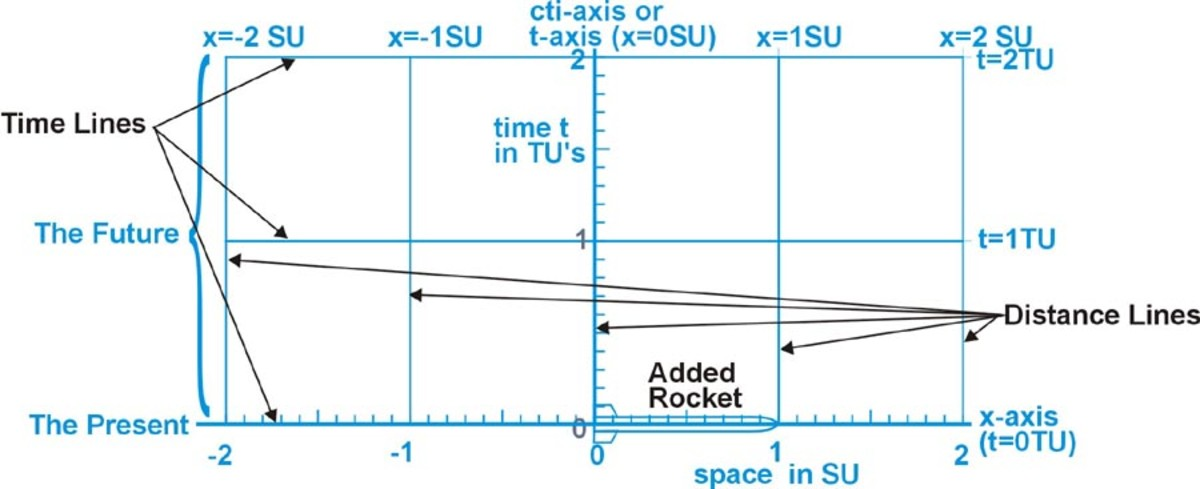Fig. 1 The prime observer's x,t coordinate system (the reference system)

## The Galilean Transformations

Before special relativity, transforming measurements from one inertial system to another system moving with a constant speed relative to the first, seemed obvious.** This was defined by the set of equations called the Galilean transformations. The Galilean transformations were named after Galileo Galilei.

Galilean Transformations* .........Inverse Galilean Transformations*

x' = x-vt ........................................x = x'+vt

y' = y ..............................................y = y'

Scroll to Continue

z' = z .............................................z = z'

t' = t ..............................................t = t'

The object is in any other inertial system that is moving through the observer's system. To compare the coordinates of this object, we plot the object's coordinates using the inverse Galilean transformations on the observer's Cartesian plane. In fig. 2 we see the observer's rectangular coordinate system in blue. The object's coordinate system is in red. This two-frame diagram compares the coordinates of the observer to the coordinates of an object moving relative to the observer. The object's rocket is one space unit long and passing the observer at a relative speed of 0.6c. In the diagram the speed v is represented by its slope (m) relative to the blue time axis. For a point on an object with a relative velocity of 0.6c to the observer would have a slope m = v/c = 0.6. The speed of light c is represented by its slope c = c/c = 1, the black diagonal line. The length of the rocket is measured as one space unit in both systems. The time units for both systems are represented by the same vertical distance on the paper.

* Modern Physics by Ronald Gautreau & William Savin (Schaum's Outline Series) ** Concepts of Modern Physics by Arthur Beiser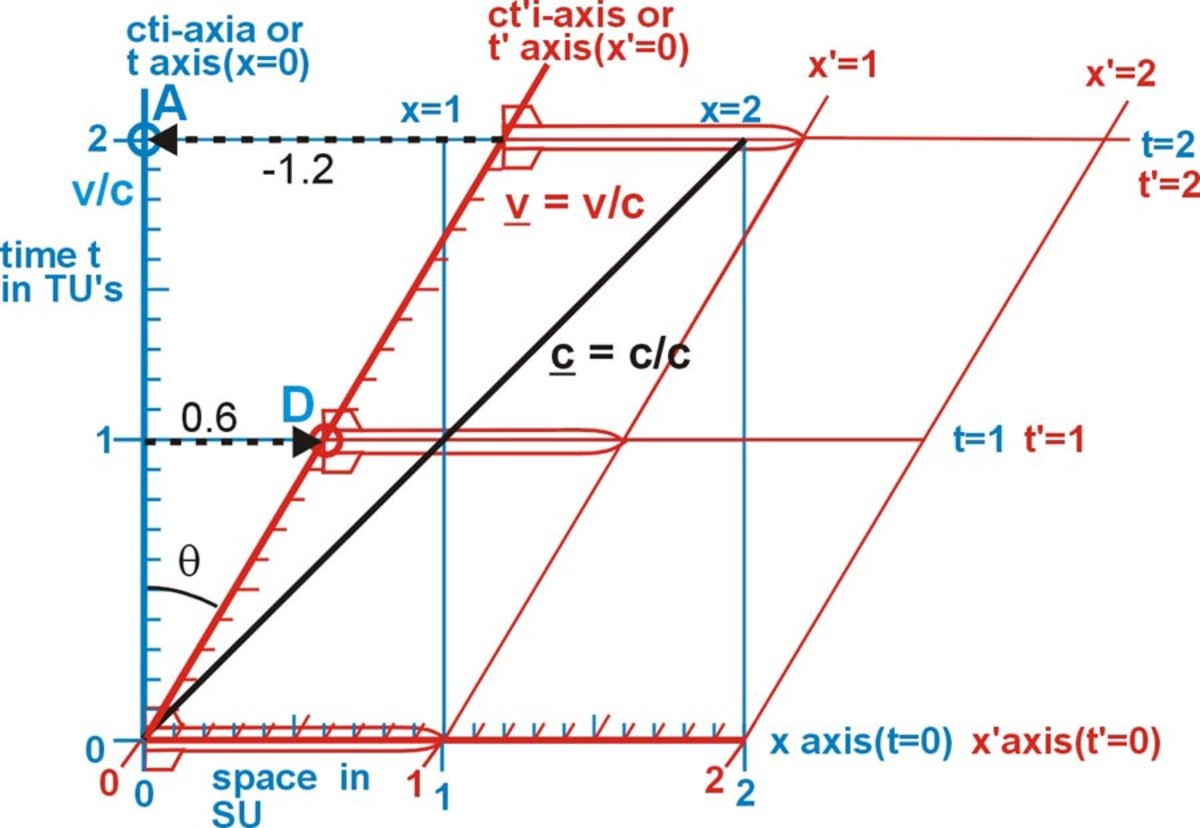Fig. 2 A two frame diagram showing Galilean transformations for a relative speed of 0.6c

## The Lorentz Transformations

The Lorentz transformations are a cornerstone in the Special Theory of Relativity. This set of equations enables electromagnetic quantities in one frame of reference to be transformed into their values in another frame of reference moving relative to the first. They were found by Hendrik Lorentz in 1895.** These equations can be used on any objects, not just electromagnetic fields. By holding the velocity at a constant and using the inverse Lorentz transformations x' and t', we can plot the object's coordinate system on the observer's Cartesian plane. See figure 3. The Blue coordinate system is the observer's system. The red lines represent the coordinate system of the object (the system that is moving relative to the observer).

Lorentz transformations* .........Inverse Lorentz transformations*

x' = (x-vt)/(1-v2/c2)1/2 ......................x = (x'+vt')/(1-v2/c2)1/2

y' = y ...........................................y = y'

z' = z........................................... z = z'

t' = (t + vx/c2)/ (1-v2/c2)1/2 .......t = (t' - vx'/c2)/ (1-v2/c2)1/2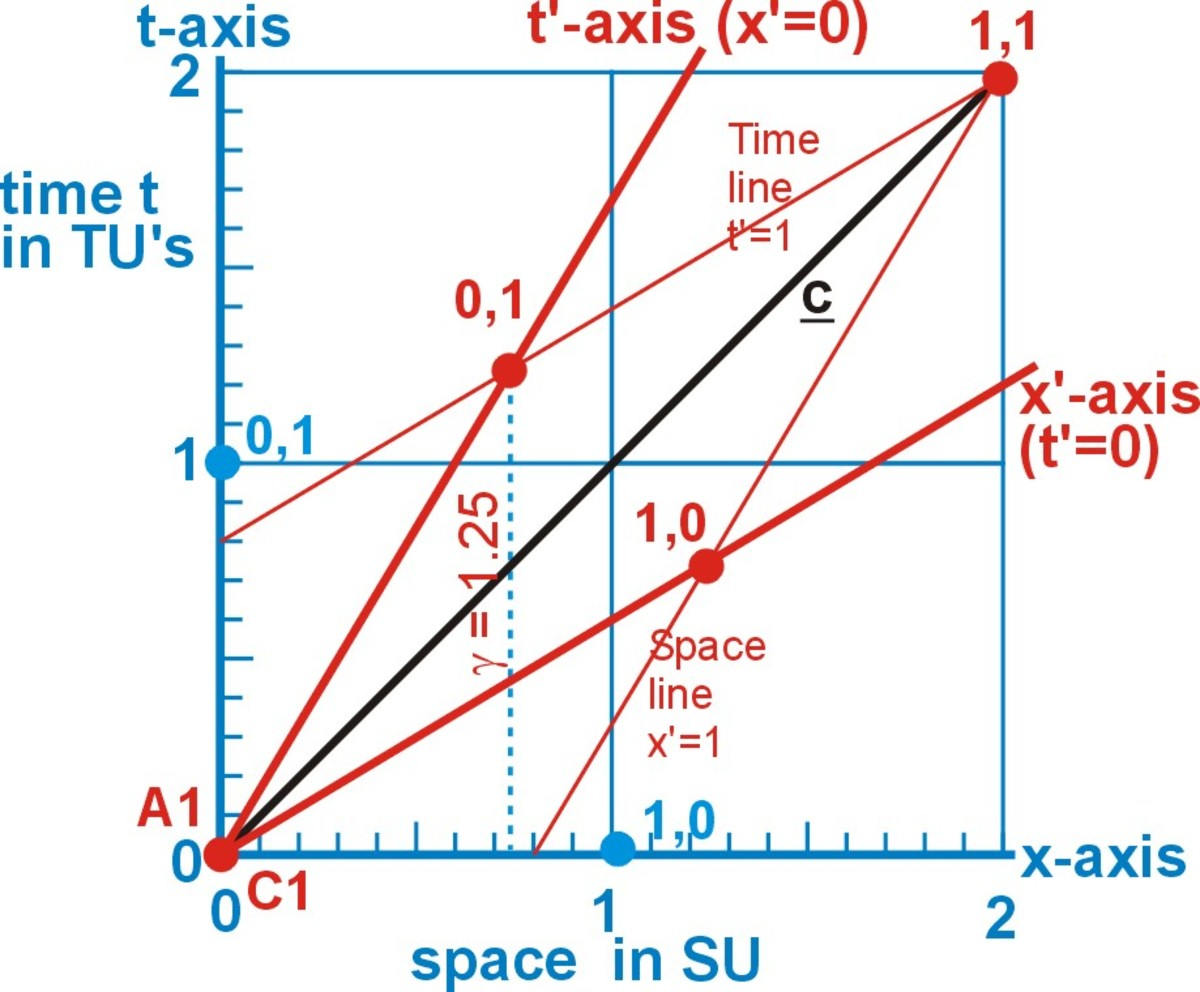Fig 3 Plotting points of the object’s coordinates on the observer’s space-time diagram produces a two frame diagram called the x,t Minkowski diagram.***

In fig. 3 to plot some of the key points of the object's coordinates use the inverse Lorentz transformations on the observer's space-time diagram. Here the object has a relative speed of 0.6c to the observer and

the relativity factor γ (gamma) = 1/(1-v2/c2) ½ = 1.25.

That is to the observer, the object's one time unit 0,1 occurs 0.25 time units later than his on time unit 0,1. By connecting the points with straight lines that extend to the edge of the observers plane, we produce the coordinate system of the object, relative to the observer's coordinate system. We can see the coordinates 0,1 and 1,0 in the object's system (red) are in a different position than the same coordinates in the observer's system (blue).

** Concepts of Modern Physics by Arthur Beiser

***A similar but simpler x,t Minkowski diagram was in Space-time Physics by E.F. Taylor & J.A. Wheeler

## The Minkowski Diagram

The results of plotting the x,t points and lines determined by the equations of the Lorentz transformations is a 2-D, x,t Minkowski space-time diagram (fig 4). This is a two-frame or two-coordinate diagram. The observer's time axis t represents the observer's path through time and space. The object is moving to the right past the observer with a speed of 0.6c. This diagram compares the relative speed (v) between the object and the observer to the speed of light (c). The slope or tangent of the angle (θ) between the axes (t and t' or x and x') is the ratio v/c. When an object has a relative velocity to the observer of 0.6c, the angle θ between the observer's axis and the objects axis, is θ = arctan 0.6 = 30.96O.

In the diagrams below I have added scales (1/10th unit) to the t' and x' axes. Notice, both the object's time and spatial scales are of equal lengths. These lengths are greater then the lengths of the observer's scales. I added rockets to fig. 4 at different positions in time. A is the observer's rocket (in blue) and B is the object's rocket (in red). Rocket B is passing rocket A with a speed of 0.6c

Most important, both systems will measure the speed of light as the value of one space unit divided by one time unit. In fig. 5 both rockets would see light (the black line) move from the rocket's tail at the origin to its nose, at 1SU Space unit) in 1TU (time unit). And in fig 5 we see light emitted in all directions from the origin, at time equals zero. After one time unit the light would have traveled one space unit (S'U) in both directions from either time axis.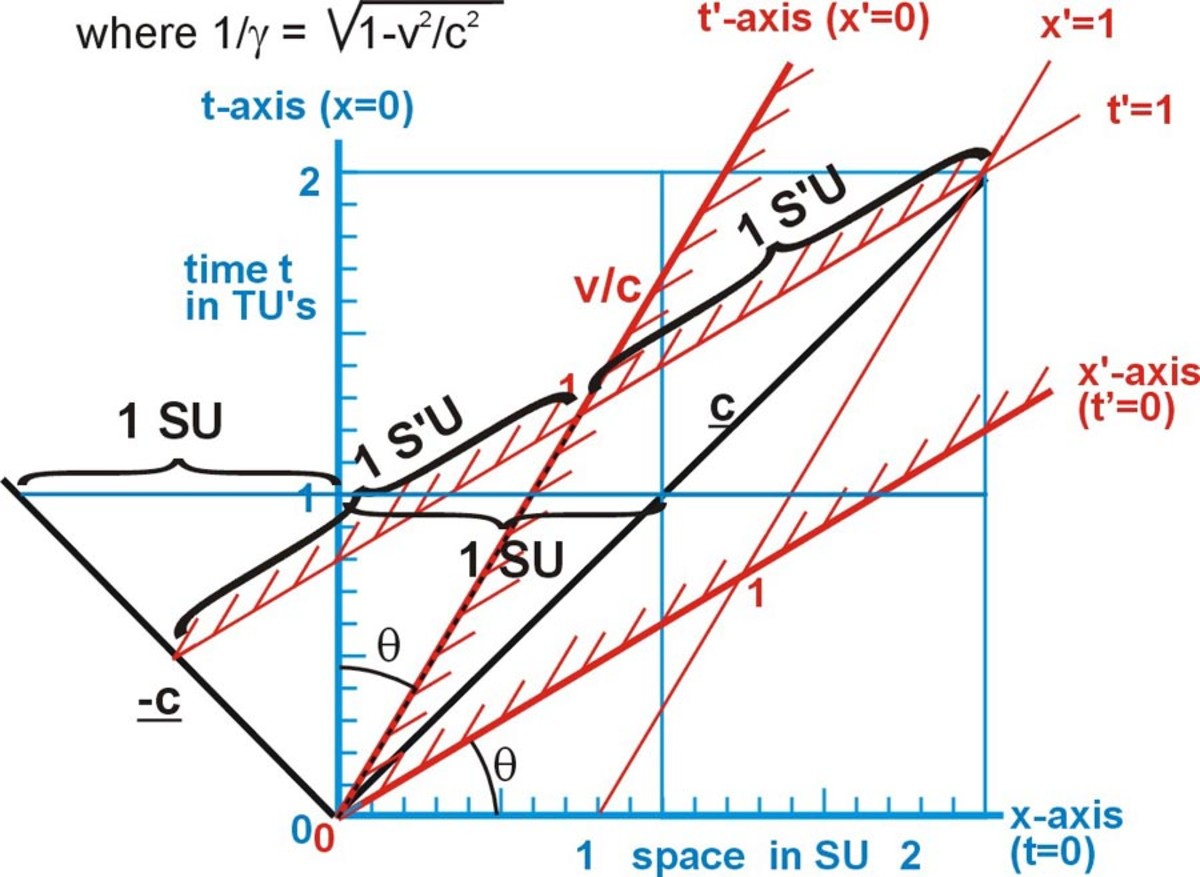Fig. 5 The speed of light is the same in both systems

## An Invariant

An invariant is the property of a physical quantity or physical law of being unchanged by certain transformations or operations. Things which are the same for all frames of reference are invariant. When an observer is not accelerating, and he measures his own time unit, space unit, or mass, these remain the same (invariant) to him, regardless of his relative velocity between the observer and other observers. Both of the postulates of the special theory of relativity are about invariance.

## The Hyperbola of Invariance

To draw the Minkowski diagram we held the velocity constant and plotted different x,t coordinates using the inverse Lorentz transformations. If we plot a single coordinate at many different velocities using the inverse Lorentz transformations, it will trace a hyperbola on the diagram. This is the hyperbola of invariance because every point on the curve is the same coordinate for the object at a different relative velocity to the observer. The upper branch of the hyperbola in fig. 6 is the locus of all the points for the same time interval the object, at any velocity. To draw this we will use the inverse Lorentz transformations to plot the point P' (x',t'), where x' = 0 and t' = 1. This is one of the object's time units on its time axis. If we were to plot this point on the x,t Minkowski diagram, as the relative speed between this point and the observer increases from -c to almost c, it would draw the upper branch of a hyperbola. The distance S from the origin to the point P where the observer's time axis (cti) crosses this hyperbola is the observer's one time unit. The distance S' from the origin to the point where the object's time axis (ct'i) crosses this hyperbola is the object's one time unit. Since the distance to both these points is one time interval, they are said to be invariant. See fig. 7. Plotting the point (0',-1') for all possible velocities will produce the lower branch of this same hyperbola. The equation of this hyperbola is

t2-x2 = 1 or t = (x2 + 1) 1/2.

Table 1 calculates the x position and the time t for the point x'=0 and t'=1 of the object moving past the observer at several different velocities. This table also shows the invariant. That for every different velocity

S'2 = x'2-t'2 = -1.

Thus the square root of S'2 is i for every velocity. The x,t points from the table are plotted on fig. 1-8 as small red circles. These points are used to draw the hyperbola.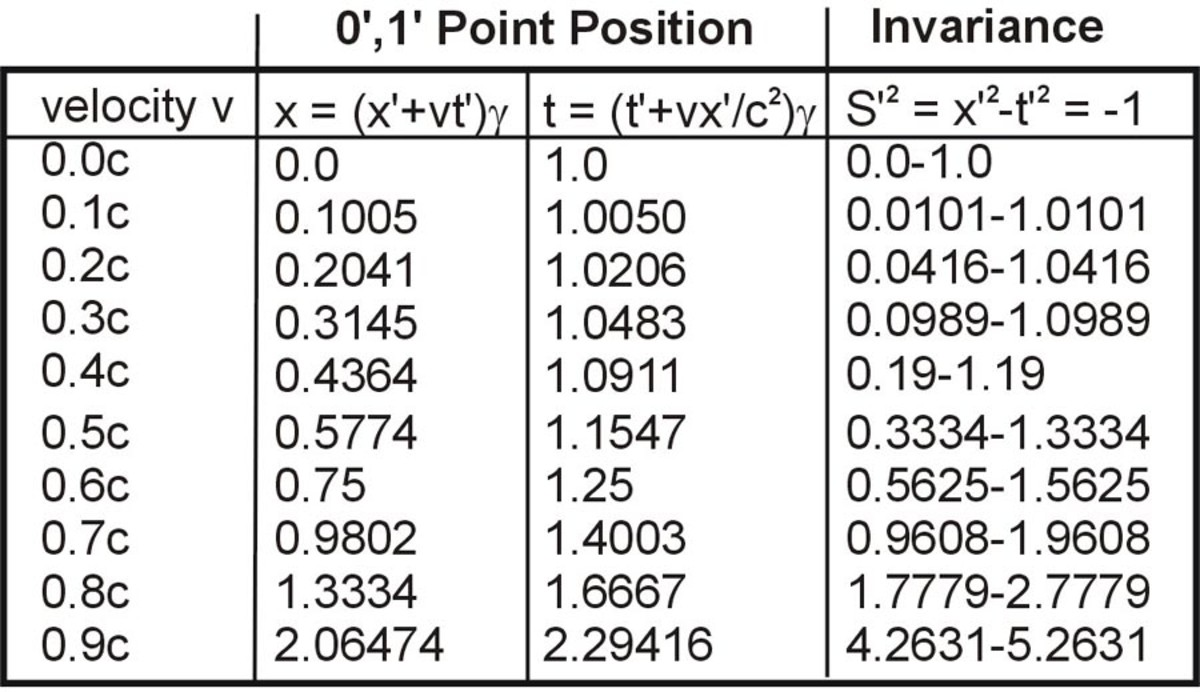Table 1 The positions of points in the first quadrant for point P (0,1) in the hyperbola t = (x2+1)½

Plotting the points (1',0') and (-1',0') for all possible velocities, will produce the right and left branch of the hyperbola x2-t2 = 1 or t = (x2-1) 1/2, for the space interval. This is illustrated in fig. 7. These can be called the hyperbolas of invariance. Each different point on a hyperbola of invariance is the same coordinate for the object (x',t'), but at a different speed relative to the observer.

## The Hyperbola of Invariance for Different Time Intervals

The inverse Lorentz transformations for x and t are x = (x'+vt')/(1-v2/c2)1/2 and t = (t' - vx'/c2)/ (1-v2/c2)1/2.

For the object's t'-axis, x' = 0 and the equations become x = (vt')/(1-v2/c2)1/2 and t = (t'/ (1-v2/c2)1/2. If we plot these equations for several values of t' it will draw a hyperbola for each different value of t'.

Fig. 7a shows 5 hyperbolas all plotted from the equation ((x2 + t2)½)/(1-v2/c2)1/2. The hyperbola T'=0.5, represents where the object's coordinate point (0,0.5) might be located in the observer's coordinate system. That is each point in the hyperbola represents the object's point (0,0.5) at a different relative speed between the object and the observer. The hyperbola T'=1 represents the location of the object's point (0,1) at all possible relative speeds. The hyperbola T'=2 represents the point (0,2) and so on with the others.

Point P1 is the position of the object's coodinate (0,2) that has a relative speed of -0.8c to the observer. The speed is negative because the object is moving to the left. Point P2 is the position of the object's coordinate (0,1) that has a relative speed of 0.6c to the observer.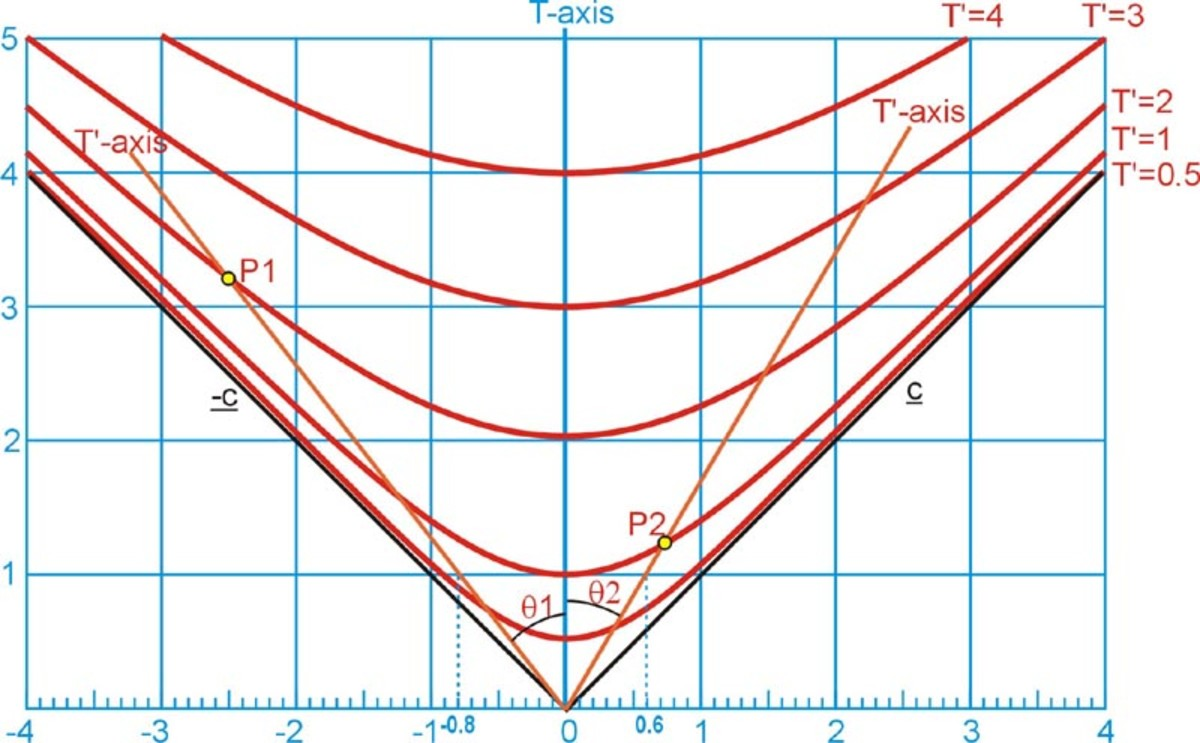Fig. 7a SomeTime Hyperbolas of invariance for different vales of T’

## The Invariance of the Interval

An interval is the time separating two events, or the distance between two objects. In fig. 8 & 9 the distance from the origin to a point in 4-dimensional space-time is the square root of D2 = x2 + y2 + z2 + (cti) 2. Since i2 = -1 the interval becomes the square root of S2 = x2 + y2 + z2 - (ct) 2. The Invariance of the interval can be expressed as S2 = x2 + y2 + z2 - (ct) 2 = S'2 = x'2 + y'2 + z'2 - (ct') 2. For the invariant of the interval in the x,t Minkowski diagram is S2 = x2 - (ct)2 = S'2 = x'2 - (ct') 2 . This means that the interval to a point (x,t) on the x or t axis, in the observer's system, measured in observer units, is the same interval to the same point (x',t') on the x' or t' axis, measured in the objects units. In figure 8 the Hyperbola equation ±cti = (x2-(Si) 2)1/2 and in figure 8a the Hyperbola equation ±cti = (x2-(Si) 2)1/2. Thus these equations using the distance to a point S' can be used to plot the hyperbola of invariance on the Minkowski diagram.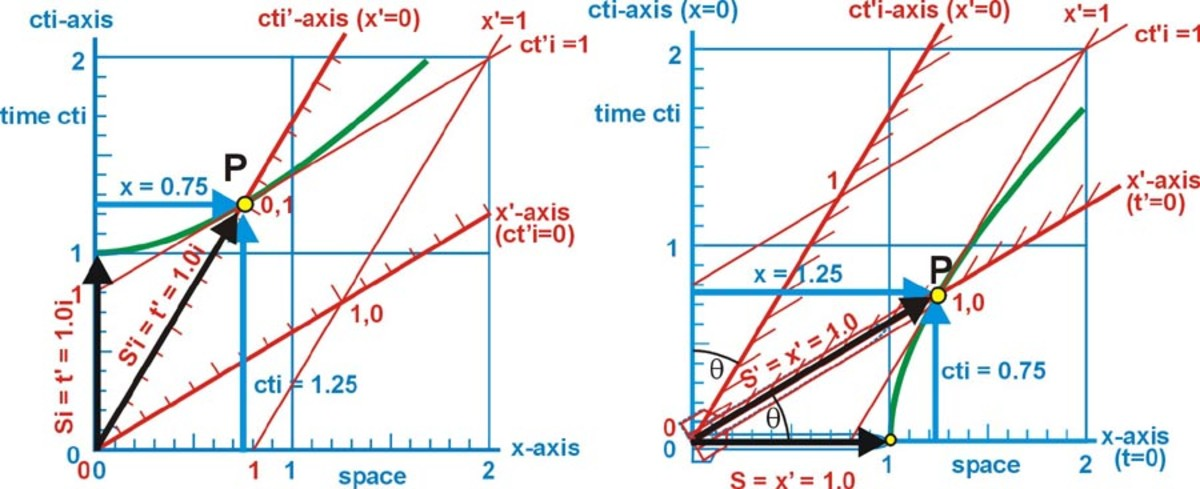Fig. 8 The invariant time interval ......... Fig. 8a The invariant space interval

## Using the Cone of Light as a 3rd Way of Visualizing the Hyperbola of Invariance

In fig. 9 a light is emitted at point P1 (0,1) on the observer's x,y plane at t = 0. This light will travel out from this point as an expanding circle on the x,y plane. As the expanding circle of light moves through time it traces out a cone of light in space-time. It will take one time unit for the light from P1 to reach the observer at point 0,1 on the observer's x,t plane. This is where the cone light just touches the observer's x,y plane. However, the light will not reach a point that 0.75 units along the x-axis until another 0.25 time units have pasted. This will occur at P3 (0.75,1.25) on the observer's x,t plane. By this time the intersection of cone of light with observer's x,y plane is a hyperbola. This is the same hyperbola as plotted using the inverse Lorentz transformation and as determined by using the invariance of the interval.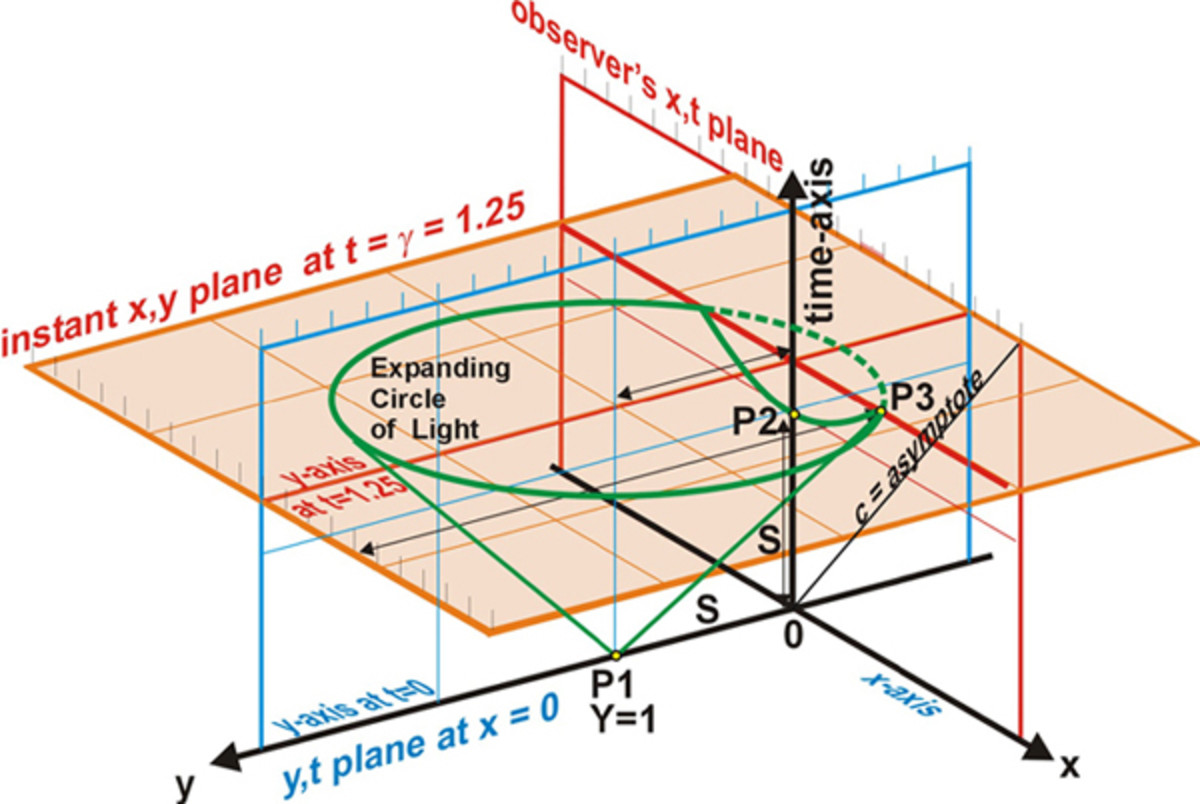Fig. 9 The intersection of the cone of light with the observer’s x,t plane

## The Scale Ratio 

In fig. 10 the rocket B has a relative velocity of 0.6c to rocket A. We see that the distances representing one space unit and one time unit for rocket B are longer than the distances representing one space unit and one time unit for rocket A. The scale ratio for this diagram is the ratio between these two different lengths. We see a horizontal dotted line passing through the one time unit on the objects t'-axis passes through the observer's t axis at γ = 1.25 uints. This is the time dilation. That is, to the observer time is moving slower in the object's system than his time, by the factor γ = 1/(1-(v/c)2)½. The distance the object would travel during this time is γv/c = 0.75 space units. These two dimensions determine the scale on the object's axis. The ratio between the units of the scales (t/t') is represented by the Greek letter sigma σ and

σ = ((γ)2 +(γ(v/c)) 2)1/2. The scale ratio σ

For a speed of 0.6c, σ = (1.252 + 0.752) 1/2 = 1.457738. This is the hypotenuse of the triangle whose sides are γ and γv/c. These are indicated by the dotted black lines in fig. 10. Also we see the arc of a circle crosses the t'-axis at t' = 1 time unit, and it crosses the t-axis at t = 1.457738 time units. The scale ratio s increases as the speed between the object and the observer increases.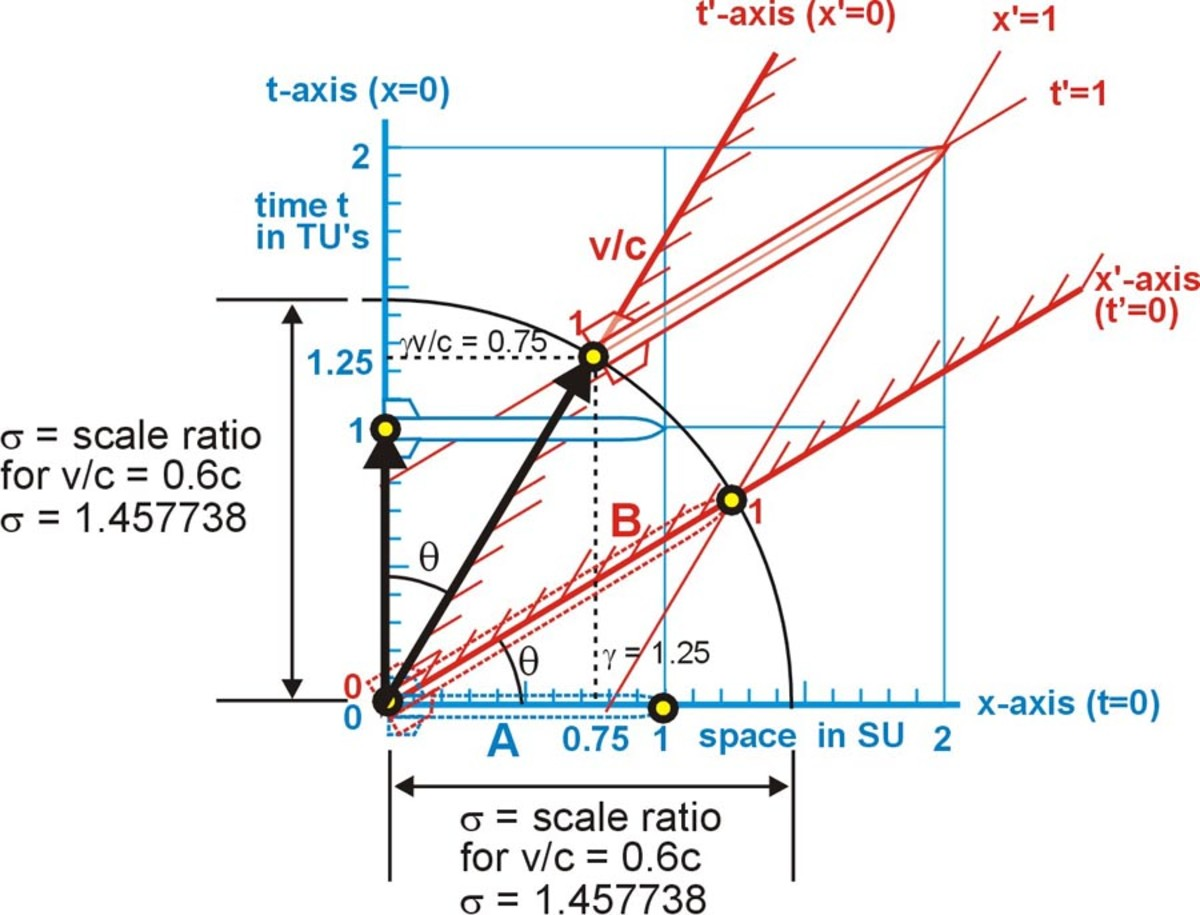Fig. 10 The scale ratio, compares the lengths of the same units in both systems

## The Line of Simultaneity (A Time Line)

A line of simultaneity is a line on the diagram, where the whole length of the line represents one instant in time. In fig. 11 the lines of simultaneity (dotted black lines) for the observer, are any lines on the space-time diagram that are parallel to the observer's spatial axis (a horizontal line). The observer measures his own rocket's length along one of his lines of simultaneity as one space unit long. In fig. 12 the lines of simultaneity are also shown as black dashed lines that are parallel to the object's space axis. Each line represents the same time increment, from one end to the other, for the object. The object measures the length of his rocket as one space unit along one of his lines of simultaneity. All lengths in the coordinate system are measured along one or another of these lines. And all time measurements are indicted by the distance of this line from its spatial axis.

In fig. 12 the object has a relative speed of 0.6c to the observer. The object's rocket is still one space unit long but on the diagram it appears as stretched out through space and time, by s (the scale ratio). The observer will measure the length of object's rocket along one of the observer's lines of simultaneity (the orange dotted lines). Here we will use the observer's space axis as the line of simultaneity. Therefore, the observer will measure the length of the object's rocket (when t =0) from the nose of rocket B1 at t' = -0.6TU to the tail of rocket B2 at t' = 0.0 (its length at one instant in his time). Thus the observer will measure the length of the object's rocket as contracted to 0.8 its original length on his line of simultaneity. The images of instant sections of the objects rocket that were emitted at different times all arrive at the eye of the observer at the same instant.

In fig. 11 we see the observer's lines of simultaneity. At t = 0, a light is flashed at the front and rear of the observer's rocket. The black lines representing the speed of light is at a 45O angle on the x,t Minkowski diagram. The rocket is one space unit long and the observer is at the mid point of the rocket. The light from both flashes (represented by the solid black lines) will arrive at observer at the same time (simultaneously) at t = 0.5. In fig. 12 the object's rocket is moving relative to the observer with a speed of 0.6c. A secondary observer (B) is at the midpoint on the object's rocket. A light is flashed at the front and rear of the object's rocket at the same instant relative to B. The light from both flashes (represented by the solid black lines) will arrive at the object's observer (B) at the same time (simultaneously) at t' = 0.5.

We have seen a brief summary of the Special Theory of Relativity. We developed the Prime Observer's coordinate system and the Secondary Observer's (the object's) coordinate system. We examined the two-frame Diagrams, with the Galilean Transformations and the Lorentz Transformations. The development of the x,y Minkowski diagram. How the hyperbola of invariance is created by the sweep of a point on the T' axis for all possible speeds, in the x,t Minkowski diagram. Another hyperbola is swept out by a point on the X' axis. We examined the scale ratio s and the line of simultaneity (a time line).

Lucy on January 09, 2016:

Thank you so much! I have been struggling to find any good resources and getting very confused while learning special relativity, this was really helpful!

Daniel on May 12, 2013:

What spectacular work. Truly astounding Minkowski Diagram illustrations. I will make sure my children and grandchildren study and memorize these.

Phys. on March 16, 2012:

Great! :)